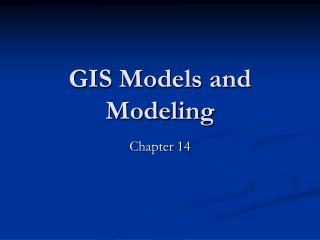DownloadDownload PresentationGIS Models and Modeling

# GIS Models and Modeling

Download Presentation## GIS Models and Modeling

- - - - - - - - - - - - - - - - - - - - - - - - - - - E N D - - - - - - - - - - - - - - - - - - - - - - - - - - -
##### Presentation Transcript

1. GIS Models and Modeling Chapter 14

2. Introduction • A model is a simplified representation of a phenomenon or system • GIS modeling is as the use of GIS in the process of building models with spatial data. • spatial data • use of modeling • discipline specific

3. GIS Modeling • GIS assists modeling • Integration of different sources then able to link and display together. • Vector or raster? • Raster: continuous, remotely sensed • Vector: well defined locations and shapes or network. • Can be integration of vector and raster. • Just GIS or linked to other packages?

4. Three Scenarios • Loose coupling • transfer of files to other programs • export • example statistical packages • Tight coupling • common user interface or menu selection to call • Embedded system • menus to call subsystems (ESRI products)

5. Binary models • A binary model uses logical expressions to select map features from a composite map or multiple grids. • Map overlay to prepare for vector query • Multiple grid process for raster • Siting common application with multiple criteria (example Conservation Reserve Program)

6. Binary models • Binary models can be used for change detection. • Sometimes binary models are used at the beginning stage of modeling.

7. Index Models • An index model used the index value calculated from a composite map or multiple grids to produced a ranked map. • Selected variables are evaluated at two levels • Relative importance, assigning a weight • Observed values are evaluated and given scores. • ArcView Model Builder • Suitability and vulnerability analysis

8. Regression Models • Regression model relates a dependent variable to a number of independent variables in an equation. • Linear regression is used when the dependent variables and independent variables are all numeric. • Logistic regression is used when the dependent variable is a binary phenomenon and the independent variables are categorical or numeric.

9. Process Models • A process model integrates existing knowledge about the environmental processes in the real world into a set of relationships and equations for quantifying the process. • Universal Soil Loss Equation (USLE) • Agricultural Nonpoint Source (AGNPS) • Soil and Water Assessment Tool (SWAT) • Typically raster-based • Most commercial GIS do not offer.